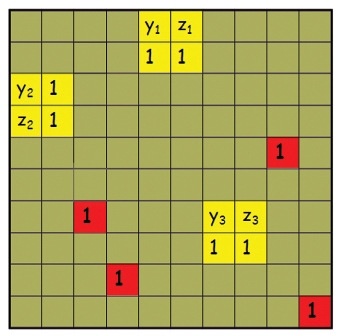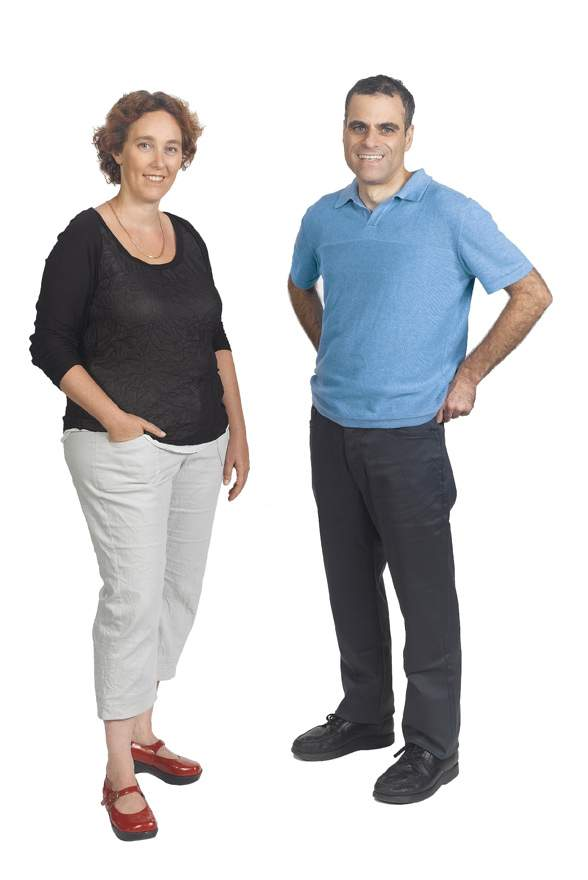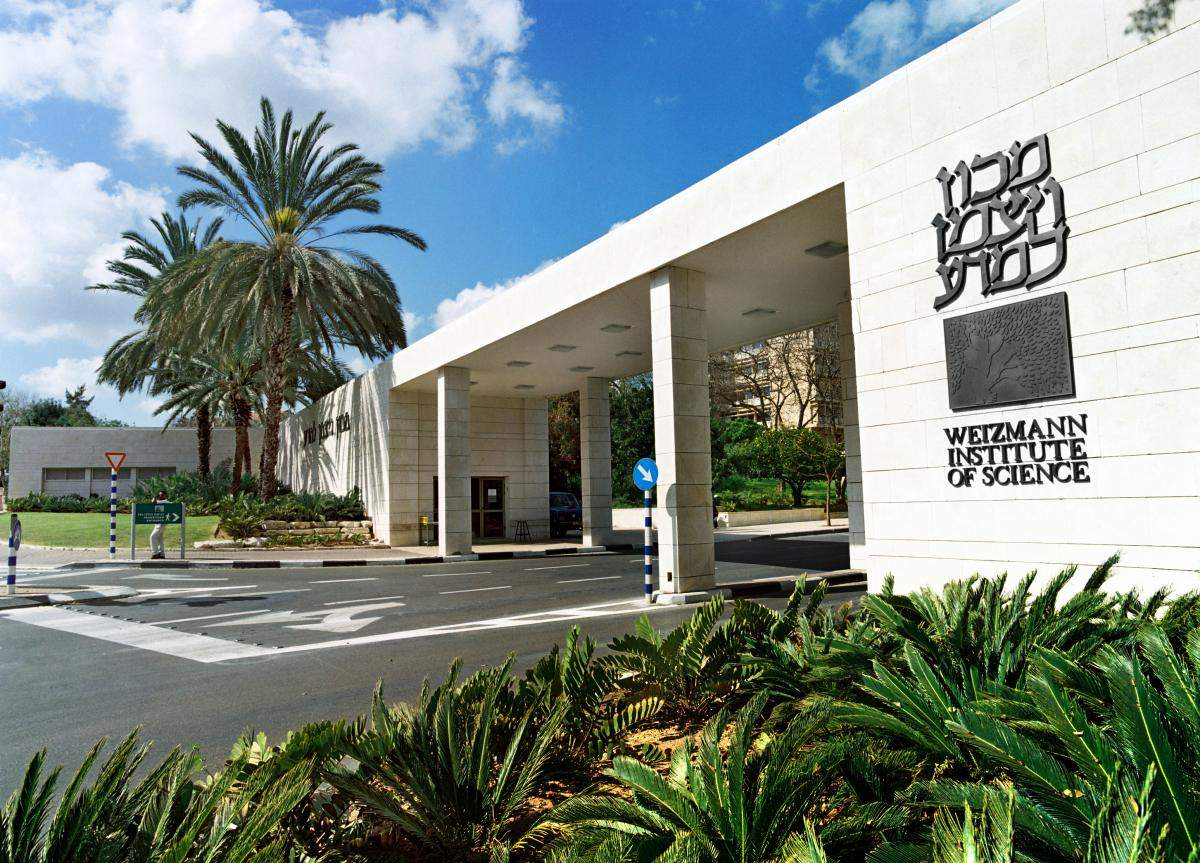# Are There Limits to Computing?

## You are here

### TagsWhat is the meaning of the Universe? A thousand years from now, our computers still will not be answering such questions. Other types of questions are theoretically answerable, but they may require an impossible amount of resources in practice. Where, then, is the dividing line between the possible and the impossible in computation?

To find out, researchers from around the globe, including scientists in the Weizmann Institute’s Faculty of Mathematics and Computer Science, try to figure out the resources needed to solve particular problems. A problem is considered solvable, for instance, if the amount of processing it requires is linear (or even squared) in relation to the length of the input. But when the computation process grows exponentially in proportion to the data, it soon becomes unsolvable in practical terms.

According to Prof. Ran Raz, one way to begin the search for the dividing line is based on the consideration of “weakened” or “simplified” models for solving various problems. These might give researchers tools to advance, step by step, toward an understanding of more general models for solving problems.

Prof. Irit Dinur tests questions that cannot, as yet, be answered. If any solutions were to be found, however, her research would help us decide whether they are correct. (When we verify a solution – even one that is not ours – we verify that the problem can be solved.) She develops algorithms that represent the solution in such a way that its veracity can be tested through checking a small number of its elements. Thus each piece of the new representation contains information on the correctness of the entire solution.Prof. Ran Raz is Director of the Moross Research School of Mathematics and Computer Science.

### Tags: